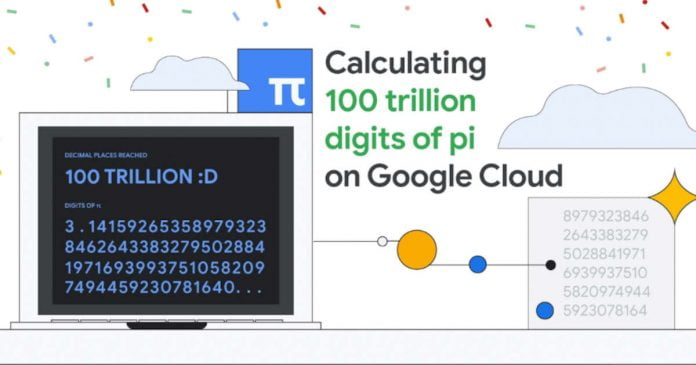Emma Haruka Iwao, one of the developers of the Google Cloud service, and her colleagues said that they calculated the number of Pi to a new record number of digits. According to Iwao, the team calculated the mathematical constant to 100 trillion decimal places.

The most recent record was 62.8 trillion digits, set by a team from the Graubünden University of Applied Sciences in Switzerland.

Pi is a mathematical constant that describes the ratio of a circle’s circumference to its diameter. It is an irrational number, which means it has an infinite number of non-repeating decimal places. Pi has been known since ancient times, and mathematicians have been trying to calculate the number ever since.

Google engineers used a cloud system that used a total of 128 processors, 864 GB of RAM and 554 TB of disk space. Even with such considerable powers, the calculation of the number Pi up to 100 trillion digits took a long time — 157 days, 23 hours and 31 minutes. For the current record, 82,000 terabytes of data were processed.

Iwao also noted that it would take more than 3.1 million years to read all 100 trillion digits aloud, one digit per second. And in case you’re wondering, the 100th trillion decimal place of Pi is zero.

This site uses Akismet to reduce spam. Learn how your comment data is processed.